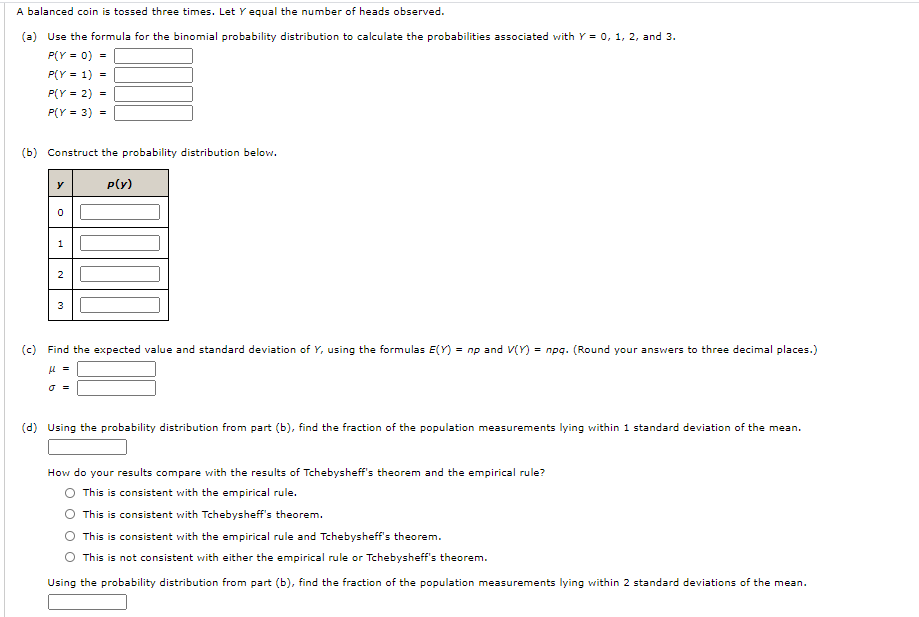# Question Solved1 AnswerA balanced coin is tossed three times. Let Y equal the number of heads observed. (a) Use the formula for the binomial probability distribution to calculate the probabilities associated with Y = 0, 1, 2, and 3. PCY - 0) = P(Y = 1) = P(Y = 2) = P(Y = 3) - (b) Construct the probability distribution below. y ply) 0 1 2 3 (c) Find the expected value and standard deviation of Y, using the formulas E(Y) = np and ViY) = npg. (Round your answers to three decimal places.) u = (d) Using the probability distribution from part (b), find the fraction of the population measurements lying within 1 standard deviation of the mean. How do your results compare with the results of Tchebysheff's theorem and the empirical rule? This is consistent with the empirical rule. This is consistent with Tchebysheff's theorem. This is consistent with the empirical rule and Tchebysheff's theorem. This is not consistent with either the empirical rule or Tchebysheff's theorem. Using the probability distribution from part (b), find the fraction of the population measurements lying within 2 standard deviations of the mean. How do your results compare with the results of Tchebysheff's theorem and the empirical rule? This is consistent with the empirical rule. This is consistent with Tchebysheff's theorem. This is consistent with the empirical rule and Tchebysheff's theorem. This is not consistent with either the empirical rule or Tchebysheff's theorem.GS6IAA The Asker · Probability and StatisticsTranscribed Image Text: A balanced coin is tossed three times. Let Y equal the number of heads observed. (a) Use the formula for the binomial probability distribution to calculate the probabilities associated with Y = 0, 1, 2, and 3. PCY - 0) = P(Y = 1) = P(Y = 2) = P(Y = 3) - (b) Construct the probability distribution below. y ply) 0 1 2 3 (c) Find the expected value and standard deviation of Y, using the formulas E(Y) = np and ViY) = npg. (Round your answers to three decimal places.) u = (d) Using the probability distribution from part (b), find the fraction of the population measurements lying within 1 standard deviation of the mean. How do your results compare with the results of Tchebysheff's theorem and the empirical rule? This is consistent with the empirical rule. This is consistent with Tchebysheff's theorem. This is consistent with the empirical rule and Tchebysheff's theorem. This is not consistent with either the empirical rule or Tchebysheff's theorem. Using the probability distribution from part (b), find the fraction of the population measurements lying within 2 standard deviations of the mean. How do your results compare with the results of Tchebysheff's theorem and the empirical rule? This is consistent with the empirical rule. This is consistent with Tchebysheff's theorem. This is consistent with the empirical rule and Tchebysheff's theorem. This is not consistent with either the empirical rule or Tchebysheff's theorem.
More
Transcribed Image Text: A balanced coin is tossed three times. Let Y equal the number of heads observed. (a) Use the formula for the binomial probability distribution to calculate the probabilities associated with Y = 0, 1, 2, and 3. PCY - 0) = P(Y = 1) = P(Y = 2) = P(Y = 3) - (b) Construct the probability distribution below. y ply) 0 1 2 3 (c) Find the expected value and standard deviation of Y, using the formulas E(Y) = np and ViY) = npg. (Round your answers to three decimal places.) u = (d) Using the probability distribution from part (b), find the fraction of the population measurements lying within 1 standard deviation of the mean. How do your results compare with the results of Tchebysheff's theorem and the empirical rule? This is consistent with the empirical rule. This is consistent with Tchebysheff's theorem. This is consistent with the empirical rule and Tchebysheff's theorem. This is not consistent with either the empirical rule or Tchebysheff's theorem. Using the probability distribution from part (b), find the fraction of the population measurements lying within 2 standard deviations of the mean. How do your results compare with the results of Tchebysheff's theorem and the empirical rule? This is consistent with the empirical rule. This is consistent with Tchebysheff's theorem. This is consistent with the empirical rule and Tchebysheff's theorem. This is not consistent with either the empirical rule or Tchebysheff's theorem.